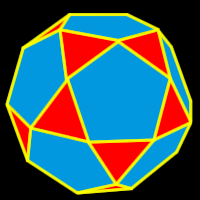Online Math Dictionary: I

Easy to understand math definitions for K-Algebra mathematics
Just scroll down or click on the word you want and I'll scroll down for you!

 icosahedron icosidodecahedron identity additive identity identity multiplicative identity imaginary number improper fraction inequality infinite infinity inscribed integer intersection intersection of sets inverse additive inverse inverse multiplicative inverse irrational number isosceles triangle isosceles trapezoid

 IcosahedronAn icosahedron is a polyhedron made up of twentyequilateral triangles.  It is one of the five Platonic Solids.      20 faces: equilateral triangles      12 vertices      30 edges      Dihedral angle: about 138.19 degrees For more info about Platonic solids, check out myPlatonic solids gallery.IcosidodecahedronAn icosidodecahedron is a polyhedron - one of the thirteen semi-regular Platonic solids.  It's created by either cutting (truncating) the dodecahedron one half of the way into each side or cutting (truncating) the icosahedron one half of the way into each side.      32 total faces: 20 equilateral triangles and                                   12 regular pentagons      30 vertices: 2 triangles and 2 pentagons      60 edges      Dihedral angle: about 142.623 degrees For more info about Platonic solids, check out myPlatonic solids gallery.